# IPv4 Address Calculation for beginners

#### Internet Protocol version 4 (IPv4)

• Example : 11000000 10101000 01100100 00000001
• Divided into 4 groups
• So each group contains 8 bits.
• To separate groups we use PERIODS
• Example : 11000000 . 10101000 . 01100100 . 00000001
• The format of IP address is dotted decimals
• We have to convert those binary bits in numbers (decimals).
• Example : 192 . 168 . 100 . 1
Starting number of each group must be 00000000 (0)
Ending number of each group must be 11111111 (255).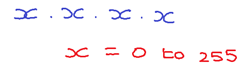Class Range Size Hosts A 1 – 126 Large 16,777,214 B 128 – 191 Medium 65,534 C 192 – 223 Small 254 D 224 – 239 Reserved E 240 – 254 Reserved
The Class D IP address is reserved for Multicast.

The IP address starting 0, 127, 255 are also reserved. The IP address starting with 127 is reserved for loopback address.

The IP address does contain 2 parts. One is Network Part and another is Host Part.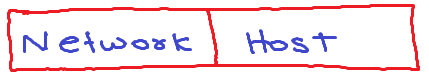But Network part and Host part will be vary with each class.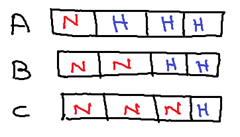Class A has 8 bits of Network Part and 24 bits of Host Part.
Class B has 16 bits of Network Part and 16 bits of Host Part.
Class C has 24 bits of Network Part and 8 bits of Host Part.

When a design a network, The Network part of all IP address must be command and host part must be unique.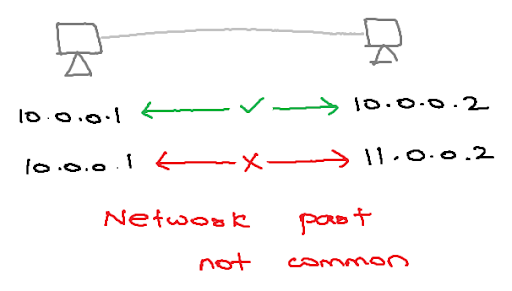Subnet Mask is used by networking devices to find out the network and host bits of IP address.
The Subnet Mask is contiguous. First Network bits and then Host bits.
For Subnet Mask: Network bits are 1’s and Host bits are 0’s

Example: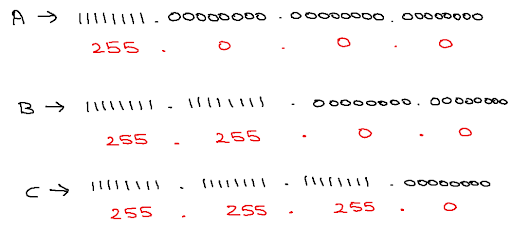#### Number of hosts:

We have a formula to find out the number of hosts in each class. And the formula is  2n – 2.
And the value of n is  number of hosts bits.
 Class A 224 – 2 16,777,214 Class B 216 – 2 65,534 Class C 28 – 2 254

In Every Network there are 2 address are reserved and that’s why we minus 2 from finding number of host addresses. One is used for Routing and we call it as Network Address and another one is used for local broadcast and we call it as Broadcast Address.

For Network Address, the network part are same as the network part IP address but the host bits of IP were 0.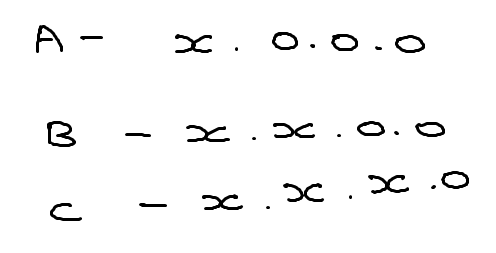For Broadcast Address, the network part are same as the network part IP address but the host bits of IP were 1.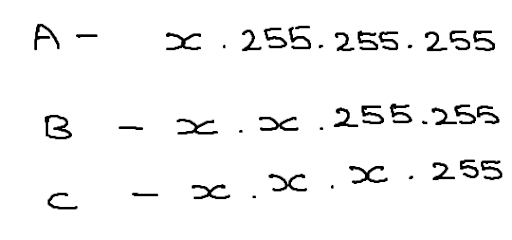Exercise 1

In this exercise we can find out subnet mask, network broadcast address, valid ip range of the network, and number of host on the network of Class C IP address.

The Above IP address belong to Class C.

Then the subnet mask is 255.255.255.0.

Ok. The first 3 groups of this IP address (not subnet mask) is host bits and the last group is host part.

Then Network Address must be : 192.168.100.0
Because all bits in the last group were 0’s : 192.168.100. 00000000
Because all bits in the last group were 1’s : 192.168.100. 11111111

The Network IP range must be from 192.168.100.1 to 192.168.100.254
Number of Host : 28 – 2 = 254.
Exercise 2

In this second exercise we can find out subnet mask, network broadcast address, valid ip range of the network, and number of host on the network of class B address.

The Above IP address belong to Class B.

Then the subnet mask is 255.255.0.0.

Ok. The first 3 groups of this IP address (not subnet mask) is host bits and the last group is host part.

Then Network Address must be : 172.16.0.0
Because all bits in the last group were 0’s : 172.16. 00000000 . 00000000
Because all bits in the last group were 1’s : 172.16. 11111111 . 11111111

The Network IP range must be from 172.16.0.1 to 172.16.255.254
Number of Host : 216 – 2 = 65534.

Exercise 2

In this second exercise we can find out subnet mask, network broadcast address, valid ip range of the network, and number of host on the network of class A address.

The Above IP address belong to Class A.

Then the subnet mask is 255.0.0.0.

Ok. The first 3 groups of this IP address (not subnet mask) is host bits and the last group is host part.

Then Network Address must be : 10.0.0.0
Because all bits in the last group were 0’s : 10. 00000000 . 00000000 . 00000000
Because all bits in the last group were 1’s : 10. 11111111 . 11111111 . 11111111

The Network IP range must be from 10.0.0.1 to 10.255.255.254
Number of Host : 28 – 2 = 16,777,254.
The End

### 2 Responses so far.

1. There is an error in last excercise.

"Number of Host : 28 – 2 = 16,777,254. "

Anyways thanks for nice tutorial it helped me a lot. :)

2. A very enlightening and descriptive guide for the beginners, it surely was a little bit hard but I really understood a lot from this post.